Published: 28 November 2019

# Analysis of vibration based windmill coupled micromachined energy harvester

Pavan R1
Shyamsundar P I2
Venkatesh K P Rao3
1, 2, 3Department of Mechanical Engineering, Birla Institute of Technology and Science, Pilani, Rajasthan, 333031, India
Corresponding Author:
Venkatesh K P Rao
Views 113

#### Abstract

The present work exploits the centripetal, Coriolis and Euler forces generated in a rotating windmill. The MEMS device is placed on the blade of a windmill to harvest the energy. Modal analysis is carried out to optimize the dimensions of the structure to match the desired conditions. The real time response of the structure and the voltage generated in the piezoelectric layer are evaluated using transient analysis. It was noticed that Euler and Coriolis forces have a significant contribution in the initial time when the wind turbine accelerates from rest. The later portion is dominated by the Coriolis and Euler forces, and in some instances they cancel out each other. However, there is always a steady contribution from the centripetal force which is proportional to the magnitude of angular velocity of the wind turbine.

## 1. Introduction

With the concerns over the depletion of fossil fuels as an energy source, renewable energy is gaining popularity. Wind energy has the potential to become a significant source of energy in the future. In , authors have given a detailed account on harnessing energy using piezoelectric materials. An idea of harnessing electricity using piezoelectric material through various devices are reported in [2-6]. This manuscript describes a new approach to increase energy production from a windmill using a MEMS energy harvester. The MEMS device consists of a proof mass coupled to four Z-shaped cantilevers. This setup is placed on the blade of a windmill.

The idea is to exploit the centripetal, Coriolis, and Euler forces generated in a rotating windmill, which otherwise goes unutilized since they increase the strain energy in the blades. This also gets subjected to random vibration, due to the wind as well as the vibrations of the whole structure. The device consists of a proof mass connected to thin beams and can move in all directions with different stiffness. When the windmill rotates, the centripetal acceleration initiates the motion in a direction. As this is a rotating frame, Coriolis force gets generated, and it acts in the direction perpendicular to rotation vector and velocity vector. These forces are supplemented by Euler forces created because of angular acceleration or deceleration of the windmill.

All these forces, along with the random vibrations, produce stresses in the beams which can be converted into electricity with the help of piezoelectric material, which otherwise goes unutilized. This can supplement the conventional wind energy produced to a reasonable extent.

Our device aims to harvest the strain energy created in the blades of the windmill with the help of a MEMS device. The device consists of a proof mass supported by four arms, which is driven by Euler, Centripetal and Coriolis forces created due to the windmill rotation and proof mass movement. This motion of the proof mass is utilized to create stress in the supporting arms, and the points of highest stress are identified. The so-identified points are made of piezoelectric materials, which are used to create potential differences and hence generate power.

Fig. 1 shows the final geometry of the energy harvester, has a uniform thickness of 5 µm. This is the optimum geometry with dimensions such that the beams are not too stiff, and the Coriolis and centripetal accelerations of the windmill rotor can cause vibrations in the proof mass, and stresses are generated in the beams so that energy can be harvested using a piezoelectric material. The material used in this study is Ga-As. Table 1 shows the geometric and material properties of the structure. The material GaAs has a Zinc blende structure with piezoelectric constant, ${d}_{14}$, 2.63 PC/N.

Table 1Material and geometric properties of the structure

 Property Value Density 5320 kg/m3 Elastic modulus 85.5 GPa Poisson’s Ratio 0.31 Length of the beam, ${l}_{b}$ 100 µm Width of the beam 5 µm Proof mass 500 × 500 µm2

Fig. 1The structure of the energy harvester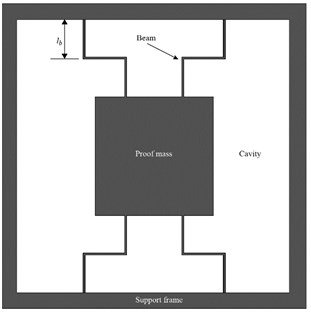## 2. Modelling and analysis

The study involves geometric parameterization, modal analysis and transient structural analysis of windmill coupled energy harvester. As the structure is of uniform thickness, a 2-D model was generated in ANSYS, and modal analysis was carried out. The proof mass length, beam thickness, and structural thickness were taken as parameters for optimization of the natural frequencies

The optimal geometry was used to analyze the stresses generated due to the action of centripetal, Coriolis and tangential forces. These stresses generate potential on the surface of the piezoelectric material. Transient structural analysis was carried out to account for the stresses induced due to the combined loading (centripetal, Coriolis and Euler forces) that can arise in the device when mounted on a rotating body such as the windmill. COMSOL Multiphysics was used to simulate this environment – The device is hinged at a distance of 88 meters from a rotating frame. This resembles the device being attached to a windmill blade, at 8 meters from the center of the rotor and is constrained from performing free motion in any direction except for rotation with respect to the center (not the proof mass). Two different profiles of angular velocity of the wind turbine were given as inputs to study the resultant stresses and piezo surface potentials generated.

A hypothetical angular velocity profile, which shows a steep and sudden increase and decrease in the angular velocities, was studied. This study was done in order to qualitatively account for the contribution of Euler force and Coriolis force in stress generation. Whereas the realistic angular velocity profile  depicting the working of a wind turbine on any normal day was taken to account for the real-life stresses produced. It represents the angular velocity profile of a wind turbine starting from rest and running at an almost constant angular velocity with mild periodic variations.

## 3. Results and discussion

### 3.1.1. Mode shapes

Using the primitive geometry (Fig. 1), modal analysis was carried out to determine the mode shapes and the corresponding natural frequencies. The modes of interest are given in Fig. 2. The four ends of the beams are fixed. Since the axial stiffness in the $Y$-direction is highest, the natural frequency of the $Y$-mode is the highest, which is evident from Fig. 2. Given these results, the modes in which various forces act are as follows: $Z$-direction – Euler, $Y$ direction – Coriolis, $X$ direction – Centripetal.

Fig. 2Mode shapes of interest: a) out of plane mode at 10.85 kHz, b) X-direction mode at 24.408 kHz, c) Y-direction mode at 30.799 kHz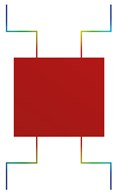a)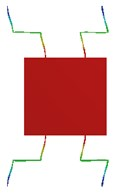b)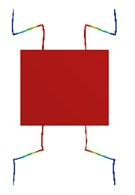c)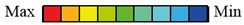Further, parametric studies were carried out to reduce the natural frequencies to the necessary limits and hence to reduce the force required to produce motion and stresses in every mode described.

### 3.1.2. Geometric parameterization

The geometrical dimensions of the structure such as the proof mass size, structure thickness, and beam thickness were chosen as parameters to optimize the natural frequencies of the structure. For this, the variation of natural frequencies with each of the aforementioned parameters is shown in Fig. 3. It can be noticed that the natural frequencies for all modes decrease with increase in the size of the proof mass, and the natural frequency for all modes increases with increase in beam thickness. It is also well established that the natural frequency increases with the increase in structure thickness.

### 3.2.1. Hypothetical profile

The maximum von mises stresses induced in the device were extracted from the transient analysis and results are as shown in Fig. 4.

The corresponding maximum surface potentials produced is compared to the input angular velocity profile and is shown in Fig. 5. It can be noticed that the Euler forces and Coriolis forces have significant contributions in the time range 0-3 seconds. This can be seen by the higher peak of the surface potential graph in this time range. The rest of the surface potential line follows the angular velocity line proportionally which implies that the centripetal force makes majority of contribution at other time ranges.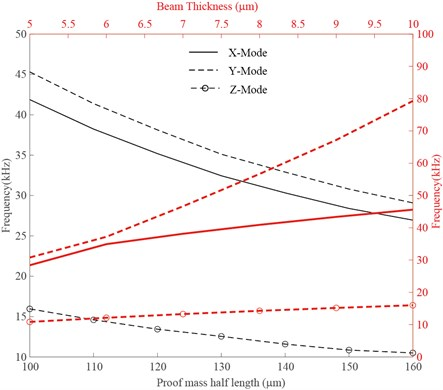Fig. 3. The variation of natural frequency with various geometric parameters

Fig. 4Maximum von mises stress developed a function of time (hypothetical profile)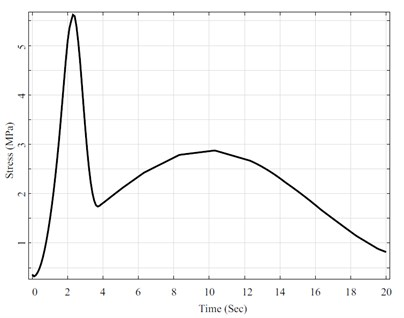Fig. 5Maximum surface potentials developed with time (hypothetical profile)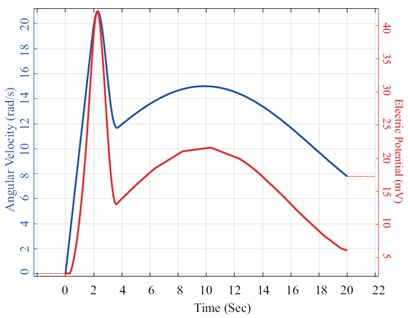### 3.2.2. Realistic profile

The maximum von mises stresses produced in the volume of the device concerning time were computed, and results are as shown in Fig. 6.

The corresponding maximum surface potentials produced is compared to the input angular velocity profile and is shown in Fig. 7. Euler forces and Coriolis forces have a significant contribution in the time range 0-5 seconds when the wind turbine accelerates from rest. After 5 seconds, when there is a mild periodic variation in the angular velocity, in some instances the Coriolis force and Euler force add up (such as the time range 10-12 seconds), and in some instances they cancel out each other (such as the time range 7-10 seconds). However, there is always a steady contribution from the centripetal force which is proportional to the magnitude of angular velocity of the wind turbine.

Fig. 6Maximum von mises stress developed with time (realistic profile)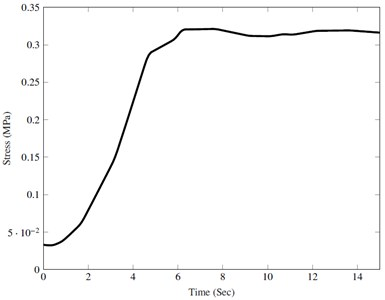Fig. 7Maximum surface potentials and angular velocity vs time (realistic profile)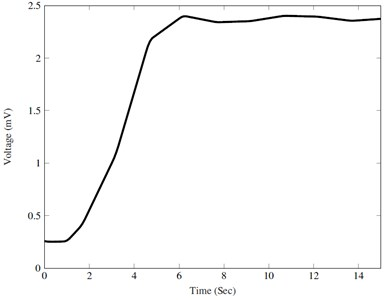## 4. Conclusions

In the present study, a new concept of harnessing wind energy is introduced. Transient analysis simulation was carried out and it was observed that potential difference of the order 1 mV develops. The mass of each device is around 10 nanograms which is negligible compared to the mass of the windmill blade and hence not affecting the basic windmill operations. When several such devices are coupled in series, significant power can be harnessed.

Future scope of the study can include experimental verification, fatigue analysis, efficiency and economic viability.